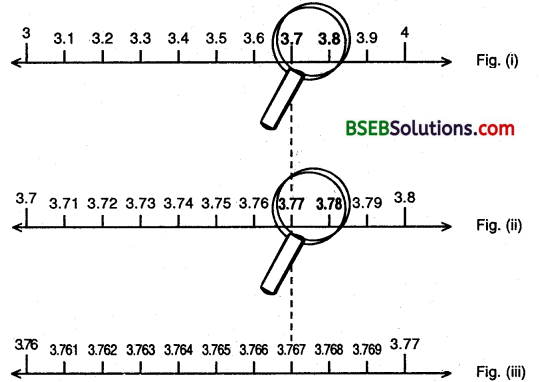# Bihar Board Class 9th Maths Solutions Chapter 1 Number Systems Ex 1.4

Bihar Board Class 9th Maths Solutions Chapter 1 Number Systems Ex 1.4 Textbook Questions and Answers.

## BSEB Bihar Board Class 9th Maths Solutions Chapter 1 Number Systems Ex 1.4Question 1.
Visualise 3.765 on the number line, using successive magnification.
Solution:
We know that 3.765 lies between 3 and 4, that is, in the interval [3, 4] to have a rough idea where it is. Now, we divide the interval [3, 4] into 10 equal parts and look at [3.7, 3.8] through a magnifying glass and realize that 3.765 lies between 3.7 and 3.8 (see figure (i). Now, we imagine that each of the new intervals [3.1, 3.2], [3.2, 3.3], …, [3.9, 4] has been sub divided into 10 equal parts. As before, we can now visualize through the magnify glass that 3.765 lies in the interval [3.76, 3.77] (see figure (ii)).So, we have seen that it is possible by sufficient successive magnifications to visualize the position (or representation of a real number with a terminating decimal expansion on the number line. Let us now try and visualize the position (or representation) of a real number with a non-terminating recurring decimal expansion on the number line. We can look at appropriate intervals through a magnifying glass and by successive magnifications visualize the position of the number on the number line.Question 2.
Visualise 4.$$\overline{26}$$ on the number line, upto 4 decimal places.
Solution:
We proceed by successive magnifications, and successively decrease the lengths of the intervals in which 4.$$\overline{26}$$ is located. 4.$$\overline{26}$$ is located in the interval [4, 5] of length 1. We further locate 4.$$\overline{26}$$ in the interval [4.2, 4.3] of length 0.1. To get more accurate visualization of the representation, we divide even this interval into 10 equal parts and use a magnifying glass to visualize that 4.$$\overline{26}$$ lies in the interval [4.26, 4.27] of length 0.01. To visualize 4.$$\overline{26}$$ in an interval of length 0.001, we again divide each of the new intervals into 10 equal parts and visualize the representation of 4.$$\overline{26}$$ in the interval [4.262, 4.263] of length 0.001. Notice that 4.$$\overline{26}$$ is located closer to 4.263 than to 4.262.

Note:
We can proceed endlessly in this manner and simultaneously imagining the decrease in the length of the interval in which 4.26 is located.